Filter
(found 5924 products)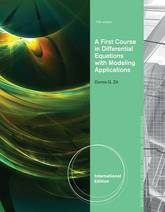A FIRST COURSE IN DIFFERENTIAL EQUATIONS WITH MODELING APPLICATIONS, 10E, International Edition strikes a balance between the analytical, qualitative, and quantitative approaches to the study of differential equations. This proven and accessible book speaks to beginning engineering and math students through a wealth of pedagogical aids, including an abundance of ...
A First Course in Differential Equations with Modeling Applications, International Edition
A FIRST COURSE IN DIFFERENTIAL EQUATIONS WITH MODELING APPLICATIONS, 10E, International Edition strikes a balance between the analytical, qualitative, and quantitative approaches to the study of differential equations. This proven and accessible book speaks to beginning engineering and math students through a wealth of pedagogical aids, including an abundance of examples, explanations, Remarks boxes, definitions, and group projects. Written in a straightforward, readable, and helpful style, the book provides a thorough treatment of boundary-value problems and partial differential equations.
245.48 USD

## A First Course in Differential Equations with Modeling Applications, International Edition

by Dennis G. Zill
PaperbackThe 10th edition of Elementary Differential Equations and Boundary Value Problems, like its predecessors, is written from the viewpoint of the applied mathematician, whose interest in differential equations may sometimes be quite theoretical, sometimes intensely practical, and often somewhere in between. The authors have sought to combine a sound and ...
Elementary Differential Equations and Boundary Value Problems
The 10th edition of Elementary Differential Equations and Boundary Value Problems, like its predecessors, is written from the viewpoint of the applied mathematician, whose interest in differential equations may sometimes be quite theoretical, sometimes intensely practical, and often somewhere in between. The authors have sought to combine a sound and accurate (but not abstract) exposition of the elementary theory of differential equations with considerable material on methods of solution, analysis, and approximation that have proved useful in a wide variety of applications. While the general structure of the book remains unchanged, some notable changes have been made to improve the clarity and readability of basic material about differential equations and their applications. In addition to expanded explanations, the 10th edition includes new problems, updated figures and examples to help motivate students. The book is written primarily for undergraduate students of mathematics, science, or engineering, who typically take a course on differential equations during their first or second year of study. The main prerequisite for reading the book is a working knowledge of calculus, gained from a normal two?(or three) semester course sequence or its equivalent. Some familiarity with matrices will also be helpful in the chapters on systems of differential equations.
177.39 USD

## Elementary Differential Equations and Boundary Value Problems

by Richard C DiPrima, William E Boyce
Paperback / softback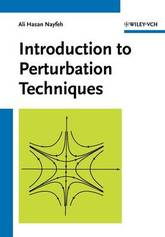Similarities, differences, advantages and limitations of perturbation techniques are pointed out concisely. The techniques are described by means of examples that consist mainly of algebraic and ordinary differential equations. Each chapter contains a number of exercises.
Introduction to Perturbation Techniques
Similarities, differences, advantages and limitations of perturbation techniques are pointed out concisely. The techniques are described by means of examples that consist mainly of algebraic and ordinary differential equations. Each chapter contains a number of exercises.
174.820000 USD

## Introduction to Perturbation Techniques

Paperback / softback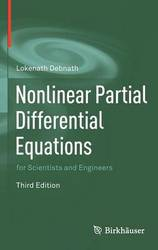The revised and enlarged third edition of this successful book presents a comprehensive and systematic treatment of linear and nonlinear partial differential equations and their varied and updated applications. In an effort to make the book more useful for a diverse readership, updated modern examples of applications are chosen from ...
Nonlinear Partial Differential Equations for Scientists and Engineers
The revised and enlarged third edition of this successful book presents a comprehensive and systematic treatment of linear and nonlinear partial differential equations and their varied and updated applications. In an effort to make the book more useful for a diverse readership, updated modern examples of applications are chosen from areas of fluid dynamics, gas dynamics, plasma physics, nonlinear dynamics, quantum mechanics, nonlinear optics, acoustics, and wave propagation. Nonlinear Partial Differential Equations for Scientists and Engineers, Third Edition, improves on an already highly complete and accessible resource for graduate students and professionals in mathematics, physics, science, and engineering. It may be used to great effect as a course textbook, research reference, or self-study guide.
146.990000 USD

## Nonlinear Partial Differential Equations for Scientists and Engineers

by Lokenath Debnath
Hardback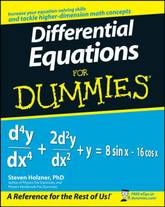The fun and easy way to understand and solve complex equations Many of the fundamental laws of physics, chemistry, biology, and economics can be formulated as differential equations. This plain-English guide explores the many applications of this mathematical tool and shows how differential equations can help us understand the world ...
Differential Equations For Dummies
The fun and easy way to understand and solve complex equations Many of the fundamental laws of physics, chemistry, biology, and economics can be formulated as differential equations. This plain-English guide explores the many applications of this mathematical tool and shows how differential equations can help us understand the world around us. Differential Equations For Dummies is the perfect companion for a college differential equations course and is an ideal supplemental resource for other calculus classes as well as science and engineering courses. It offers step-by-step techniques, practical tips, numerous exercises, and clear, concise examples to help readers improve their differential equation-solving skills and boost their test scores.
20.990000 USD

## Differential Equations For Dummies

by Steven Holzner
Paperback / softback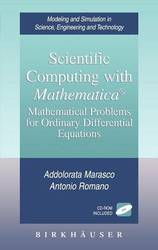Many interesting behaviors of real physical, biological, economical, and chemical systems can be described by ordinary differential equations (ODEs). Scientific Computing with Mathematica for Ordinary Differential Equations provides a general framework useful for the applications, on the conceptual aspects of the theory of ODEs, as well as a sophisticated use ...
Scientific Computing with Mathematica (R): Mathematical Problems for Ordinary Differential Equations
Many interesting behaviors of real physical, biological, economical, and chemical systems can be described by ordinary differential equations (ODEs). Scientific Computing with Mathematica for Ordinary Differential Equations provides a general framework useful for the applications, on the conceptual aspects of the theory of ODEs, as well as a sophisticated use of Mathematica software for the solutions of problems related to ODEs. In particular, a chapter is devoted to the use ODEs and Mathematica in the Dynamics of rigid bodies. Mathematical methods and scientific computation are dealt with jointly to supply a unified presentation. The main problems of ordinary differential equations such as, phase portrait, approximate solutions, periodic orbits, stability, bifurcation, and boundary problems are covered in an integrated fashion with numerous worked examples and computer program demonstrations using Mathematica. Topics and Features:*Explains how to use the Mathematica package ODE.m to support qualitative and quantitative problem solving *End-of- chapter exercise sets incorporating the use of Mathematica programs *Detailed description and explanation of the mathematical procedures underlying the programs written in Mathematica *Appendix describing the use of ten notebooks to guide the reader through all the exercises. This book is an essential text/reference for students, graduates and practitioners in applied mathematics and engineering interested in ODE's problems in both the qualitative and quantitative description of solutions with the Mathematica program. It is also suitable as a self-
157.490000 USD

## Scientific Computing with Mathematica (R): Mathematical Problems for Ordinary Differential Equations

Hardback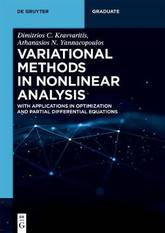This well-thought-out book covers the fundamentals of nonlinear analysis, with a particular focus on variational methods and their applications. Starting from preliminaries in functional analysis, it expands in several directions such as Banach spaces, fixed point theory, nonsmooth analysis, minimax theory, variational calculus and inequalities, critical point theory, monotone, maximal ...
Variational Methods in Nonlinear Analysis: With Applications in Optimization and Partial Differential Equations
This well-thought-out book covers the fundamentals of nonlinear analysis, with a particular focus on variational methods and their applications. Starting from preliminaries in functional analysis, it expands in several directions such as Banach spaces, fixed point theory, nonsmooth analysis, minimax theory, variational calculus and inequalities, critical point theory, monotone, maximal monotone and pseudomonotone operators, and evolution problems.
94.490000 USD

## Variational Methods in Nonlinear Analysis: With Applications in Optimization and Partial Differential Equations

by Athanasios N. Yannacopoulos, Dimitrios C Kravvaritis
Paperback / softback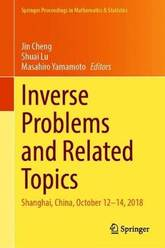This volume contains 13 chapters, which are extended versions of presentations at the International Conference on Inverse Problems at Fudan University, Shanghai, China, October 12-14, 2018, in honor of Masahiro Yamamoto on the occasion of his 60th birthday. The chapters are authored by world-renowned researchers and rising young talents, and ...
Inverse Problems and Related Topics: Shanghai, China, October 12-14, 2018
This volume contains 13 chapters, which are extended versions of presentations at the International Conference on Inverse Problems at Fudan University, Shanghai, China, October 12-14, 2018, in honor of Masahiro Yamamoto on the occasion of his 60th birthday. The chapters are authored by world-renowned researchers and rising young talents, and are updated accounts of various aspects of the research on inverse problems. The volume covers theories of inverse problems for partial differential equations, regularization methods, and related topics from control theory. This book addresses a wide audience of researchers and young post-docs and graduate students who are interested in mathematical sciences as well as mathematics.
167.990000 USD

## Inverse Problems and Related Topics: Shanghai, China, October 12-14, 2018

Hardback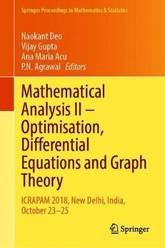This book collects original research papers and survey articles presented at the International Conference on Recent Advances in Pure and Applied Mathematics (ICRAPAM), held at Delhi Technological University, India, on 23-25 October 2018. Divided into two volumes, it discusses major topics in mathematical analysis and its applications, and demonstrates the ...
Mathematical Analysis II - Optimisation, Differential Equations and Graph Theory: ICRAPAM 2018, New Delhi, India, October 23-25
This book collects original research papers and survey articles presented at the International Conference on Recent Advances in Pure and Applied Mathematics (ICRAPAM), held at Delhi Technological University, India, on 23-25 October 2018. Divided into two volumes, it discusses major topics in mathematical analysis and its applications, and demonstrates the versatility and inherent beauty of analysis. It also shows the use of analytical techniques to solve problems and, wherever possible, derive their numerical solutions. This volume addresses major topics, such as multi-objective optimization problems, impulsive differential equations, mathematical modelling, fuzzy mathematics, graph theory, and coding theory. It is a valuable resource to students as well as researchers in mathematical sciences.
167.990000 USD

## Mathematical Analysis II - Optimisation, Differential Equations and Graph Theory: ICRAPAM 2018, New Delhi, India, October 23-25

Hardback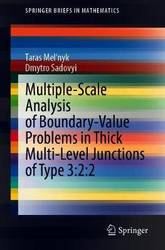This book presents asymptotic methods for boundary-value problems (linear and semilinear, elliptic and parabolic) in so-called thick multi-level junctions. These complicated structures appear in a large variety of applications. A concise and readable introduction to the topic, the book provides a full review of the literature as well as a ...
Multiple-Scale Analysis of Boundary-Value Problems in Thick Multi-Level Junctions of Type 3:2:2
This book presents asymptotic methods for boundary-value problems (linear and semilinear, elliptic and parabolic) in so-called thick multi-level junctions. These complicated structures appear in a large variety of applications. A concise and readable introduction to the topic, the book provides a full review of the literature as well as a presentation of results of the authors, including the homogenization of boundary-value problems in thick multi-level junctions with non-Lipschitz boundaries, and the construction of approximations for solutions to semilinear problems. Including end-of-chapter conclusions discussing the results and their physical interpretations, this book will be of interest to researchers and graduate students in asymptotic analysis and applied mathematics as well as to physicists, chemists and engineers interested in processes such as heat and mass transfer.
62.990000 USD

## Multiple-Scale Analysis of Boundary-Value Problems in Thick Multi-Level Junctions of Type 3:2:2

Paperback / softback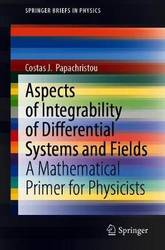This book serves as an introduction to the concept of integrability as it applies to systems of differential equations as well as to vector-valued fields. The author focuses on specific aspects of integrability that are often encountered in a variety of problems in applied mathematics, physics and engineering. The following ...
Aspects of Integrability of Differential Systems and Fields: A Mathematical Primer for Physicists
This book serves as an introduction to the concept of integrability as it applies to systems of differential equations as well as to vector-valued fields. The author focuses on specific aspects of integrability that are often encountered in a variety of problems in applied mathematics, physics and engineering. The following general cases of integrability are examined: (a) path-independence of line integrals of vector fields on the plane and in space; (b) integration of a system of ordinary differential equations by using first integrals; and (c) integrable systems of partial differential equations. Special topics include the integration of analytic functions and some elements from the geometric theory of differential systems. Certain more advanced subjects, such as Lax pairs and Backlund transformations, are also discussed. The book is written at an intermediate level for educational purposes. The presentation is as simple as the topics allow, often sacrificing mathematical rigor in favor of pedagogical efficiency.
62.990000 USD

## Aspects of Integrability of Differential Systems and Fields: A Mathematical Primer for Physicists

by Costas J. Papachristou
Paperback / softback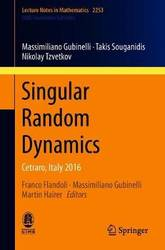Written by leading experts in an emerging field, this book offers a unique view of the theory of stochastic partial differential equations, with lectures on the stationary KPZ equation, fully nonlinear SPDEs, and random data wave equations. This subject has recently attracted a great deal of attention, partly as a ...
Singular Random Dynamics: Cetraro, Italy 2016
Written by leading experts in an emerging field, this book offers a unique view of the theory of stochastic partial differential equations, with lectures on the stationary KPZ equation, fully nonlinear SPDEs, and random data wave equations. This subject has recently attracted a great deal of attention, partly as a consequence of Martin Hairer's contributions and in particular his creation of a theory of regularity structures for SPDEs, for which he was awarded the Fields Medal in 2014. The text comprises three lectures covering: the theory of stochastic Hamilton-Jacobi equations, one of the most intriguing and rich new chapters of this subject; singular SPDEs, which are at the cutting edge of innovation in the field following the breakthroughs of regularity structures and related theories, with the KPZ equation as a central example; and the study of dispersive equations with random initial conditions, which gives new insights into classical problems and at the same time provides a surprising parallel to the theory of singular SPDEs, viewed from many different perspectives. These notes are aimed at graduate students and researchers who want to familiarize themselves with this new field, which lies at the interface between analysis and probability.
57.740000 USD

## Singular Random Dynamics: Cetraro, Italy 2016

by Nikolay Tzvetkov, Panagiotis E. Souganidis, Massimiliano Gubinelli
Paperback / softback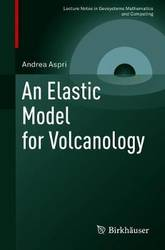This monograph presents a rigorous mathematical framework for a linear elastic model arising from volcanology that explains deformation effects generated by inflating or deflating magma chambers in the Earth's interior. From a mathematical perspective, these modeling assumptions manifest as a boundary value problem that has long been known by researchers ...
An Elastic Model for Volcanology
This monograph presents a rigorous mathematical framework for a linear elastic model arising from volcanology that explains deformation effects generated by inflating or deflating magma chambers in the Earth's interior. From a mathematical perspective, these modeling assumptions manifest as a boundary value problem that has long been known by researchers in volcanology, but has not, until now, been given a thorough mathematical treatment. This mathematical study gives an explicit formula for the solution of the boundary value problem which generalizes the few well-known, explicit solutions found in geophysics literature. Using two distinct analytical approaches-one involving weighted Sobolev spaces, and the other using single and double layer potentials-the well-posedness of the elastic model is proven. An Elastic Model for Volcanology will be of particular interest to mathematicians researching inverse problems, as well as geophysicists studying volcanology.
78.740000 USD

## An Elastic Model for Volcanology

by Andrea Aspri
Paperback / softback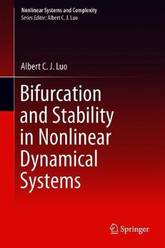This book systematically presents a fundamental theory for the local analysis of bifurcation and stability of equilibriums in nonlinear dynamical systems. Until now, one does not have any efficient way to investigate stability and bifurcation of dynamical systems with higher-order singularity equilibriums. For instance, infinite-equilibrium dynamical systems have higher-order singularity, ...
Bifurcation and Stability in Nonlinear Dynamical Systems
This book systematically presents a fundamental theory for the local analysis of bifurcation and stability of equilibriums in nonlinear dynamical systems. Until now, one does not have any efficient way to investigate stability and bifurcation of dynamical systems with higher-order singularity equilibriums. For instance, infinite-equilibrium dynamical systems have higher-order singularity, which dramatically changes dynamical behaviors and possesses the similar characteristics of discontinuous dynamical systems. The stability and bifurcation of equilibriums on the specific eigenvector are presented, and the spiral stability and Hopf bifurcation of equilibriums in nonlinear systems are presented through the Fourier series transformation. The bifurcation and stability of higher-order singularity equilibriums are presented through the (2m)th and (2m+1)th -degree polynomial systems. From local analysis, dynamics of infinite-equilibrium systems is discussed. The research on infinite-equilibrium systems will bring us to the new era of dynamical systems and control. Presents an efficient way to investigate stability and bifurcation of dynamical systems with higher-order singularity equilibriums; Discusses dynamics of infinite-equilibrium systems; Demonstrates higher-order singularity.
188.990000 USD

## Bifurcation and Stability in Nonlinear Dynamical Systems

by Albert C. J. Luo
Hardback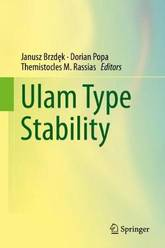This book is an outcome of two Conferences on Ulam Type Stability (CUTS) organized in 2016 (July 4-9, Cluj-Napoca, Romania) and in 2018 (October 8-13, 2018, Timisoara, Romania). It presents up-to-date insightful perspective and very resent research results on Ulam type stability of various classes of linear and nonlinear operators; ...
Ulam Type Stability
This book is an outcome of two Conferences on Ulam Type Stability (CUTS) organized in 2016 (July 4-9, Cluj-Napoca, Romania) and in 2018 (October 8-13, 2018, Timisoara, Romania). It presents up-to-date insightful perspective and very resent research results on Ulam type stability of various classes of linear and nonlinear operators; in particular on the stability of many functional equations in a single and several variables (also in the lattice environments, Orlicz spaces, quasi-b-Banach spaces, and 2-Banach spaces) and some orthogonality relations (e.g., of Birkhoff-James). A variety of approaches are presented, but a particular emphasis is given to that of fixed points, with some new fixed point results and their applications provided. Besides these several other topics are considered that are somehow related to the Ulam stability such as: invariant means, geometry of Banach function modules, queueing systems, semi-inner products and parapreseminorms, subdominant eigenvalue location of a bordered diagonal matrix and optimal forward contract design for inventory. New directions and several open problems regarding stability and non-stability concepts are included. Ideal for use as a reference or in a seminar, this book is aimed toward graduate students, scientists and engineers working in functional equations, difference equations, operator theory, functional analysis, approximation theory, optimization theory, and fixed point theory who wish to be introduced to a wide spectrum of relevant theories, methods and applications leading to interdisciplinary research. It advances the possibilities for future research through an extensive bibliography and a large spectrum of techniques, methods and applications.
125.990000 USD

## Ulam Type Stability

Hardback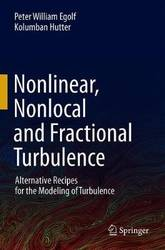Experts of fluid dynamics agree that turbulence is nonlinear and nonlocal. Because of a direct correspondence, nonlocality also implies fractionality. Fractional dynamics is the physics related to fractal (geometrical) systems and is described by fractional calculus. Up-to-present, numerous criticisms of linear and local theories of turbulence have been published. Nonlinearity ...
Nonlinear, Nonlocal and Fractional Turbulence: Alternative Recipes for the Modeling of Turbulence
Experts of fluid dynamics agree that turbulence is nonlinear and nonlocal. Because of a direct correspondence, nonlocality also implies fractionality. Fractional dynamics is the physics related to fractal (geometrical) systems and is described by fractional calculus. Up-to-present, numerous criticisms of linear and local theories of turbulence have been published. Nonlinearity has established itself quite well, but so far only a very small number of general nonlocal concepts and no concrete nonlocal turbulent flow solutions were available. This book presents the first analytical and numerical solutions of elementary turbulent flow problems, mainly based on a nonlocal closure. Considerations involve anomalous diffusion (Levy flights), fractal geometry (fractal- , bi-fractal and multi-fractal model) and fractional dynamics. Examples include a new 'law of the wall' and a generalization of Kraichnan's energy-enstrophy spectrum that is in harmony with non-extensive and non-equilibrium thermodynamics (Tsallis thermodynamics) and experiments. Furthermore, the presented theories of turbulence reveal critical and cooperative phenomena in analogy with phase transitions in other physical systems, e.g., binary fluids, para-ferromagnetic materials, etc.; the two phases of turbulence identifying the laminar streaks and coherent vorticity-rich structures. This book is intended, apart from fluids specialists, for researchers in physics, as well as applied and numerical mathematics, who would like to acquire knowledge about alternative approaches involved in the analytical and numerical treatment of turbulence.
146.990000 USD

## Nonlinear, Nonlocal and Fractional Turbulence: Alternative Recipes for the Modeling of Turbulence

by Kolumban Hutter, Peter William Egolf
Hardback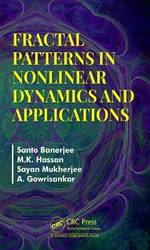Most books on fractals focus on deterministic fractals as the impact of incorporating randomness and time is almost absent. Further, most review fractals without explaining what scaling and self-similarity means. This book introduces the idea of scaling, self-similarity, scale-invariance and their role in the dimensional analysis. For the first time, ...
Fractal Patterns in Nonlinear Dynamics and Applications
Most books on fractals focus on deterministic fractals as the impact of incorporating randomness and time is almost absent. Further, most review fractals without explaining what scaling and self-similarity means. This book introduces the idea of scaling, self-similarity, scale-invariance and their role in the dimensional analysis. For the first time, fractals emphasizing mostly on stochastic fractal, and multifractals which evolves with time instead of scale-free self-similarity, are discussed. Moreover, it looks at power laws and dynamic scaling laws in some detail and provides an overview of modern statistical tools for calculating fractal dimension and multifractal spectrum.
212.01 USD

## Fractal Patterns in Nonlinear Dynamics and Applications

by Sayan Mukherjee, Santo Banerjee
Hardback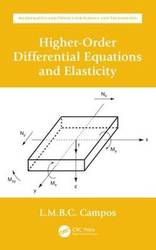Higher-Order Differential Equations and Elasticity is the third book within Ordinary Differential Equations with Applications to Trajectories and Vibrations, Six-volume Set. As a set, they are the fourth volume in the series Mathematics and Physics Applied to Science and Technology. This third book consists of two chapters (chapters 5 and ...
Higher-Order Differential Equations and Elasticity
Higher-Order Differential Equations and Elasticity is the third book within Ordinary Differential Equations with Applications to Trajectories and Vibrations, Six-volume Set. As a set, they are the fourth volume in the series Mathematics and Physics Applied to Science and Technology. This third book consists of two chapters (chapters 5 and 6 of the set). The first chapter in this book concerns non-linear differential equations of the second and higher orders. It also considers special differential equations with solutions like envelopes not included in the general integral. The methods presented include special differential equations, whose solutions include the general integral and special integrals not included in the general integral for myriad constants of integration. The methods presented include dual variables and differentials, related by Legendre transforms, that have application in thermodynamics. The second chapter concerns deformations of one (two) dimensional elastic bodies that are specified by differential equations of: (i) the second-order for non-stiff bodies like elastic strings (membranes); (ii) fourth-order for stiff bodies like bars and beams (plates). The differential equations are linear for small deformations and gradients and non-linear otherwise. The deformations for beams include bending by transverse loads and buckling by axial loads. Buckling and bending couple non-linearly for plates. The deformations depend on material properties, for example isotropic or anisotropic elastic plates, with intermediate cases such as orthotropic or pseudo-isotropic. Discusses differential equations having special integrals not contained in the general integral, like the envelope of a family of integral curves Presents differential equations of the second and higher order, including non-linear and with variable coefficients Compares relation of differentials with the principles of thermodynamics Describes deformations of non-stiff elastic bodies like strings and membranes and buckling of stiff elastic bodies like bars, beams, and plates Presents linear and non-linear waves in elastic strings, membranes, bars, beams, and plates
124.58 USD

## Higher-Order Differential Equations and Elasticity

by Luis Manuel Braga Da Costa Campos
Hardback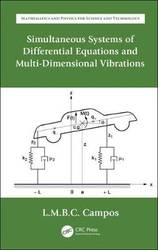Simultaneous Differential Equations and Multi-Dimensional Vibrations is the fourth book within Ordinary Differential Equations with Applications to Trajectories and Vibrations, Six-volume Set. As a set, they are the fourth volume in the series Mathematics and Physics Applied to Science and Technology. This fourth book consists of two chapters (chapters 7 ...
Simultaneous Systems of Differential Equations and Multi-Dimensional Vibrations
Simultaneous Differential Equations and Multi-Dimensional Vibrations is the fourth book within Ordinary Differential Equations with Applications to Trajectories and Vibrations, Six-volume Set. As a set, they are the fourth volume in the series Mathematics and Physics Applied to Science and Technology. This fourth book consists of two chapters (chapters 7 and 8 of the set). The first chapter concerns simultaneous systems of ordinary differential equations and focuses mostly on the cases that have a matrix of characteristic polynomials, namely linear systems with constant or homogeneous power coefficients. The method of the matrix of characteristic polynomials also applies to simultaneous systems of linear finite difference equations with constant coefficients. The second chapter considers linear multi-dimensional oscillators with any number of degrees of freedom including damping, forcing, and multiple resonance. The discrete oscillators may be extended from a finite number of degrees-of-freedom to infinite chains. The continuous oscillators correspond to waves in homogeneous or inhomogeneous media, including elastic, acoustic, electromagnetic, and water surface waves. The combination of propagation and dissipation leads to the equations of mathematical physics. Presents simultaneous systems of ordinary differential equations and their elimination for a single ordinary differential equation Includes cases with a matrix of characteristic polynomials, including simultaneous systems of linear differential and finite difference equations with constant coefficients Covers multi-dimensional oscillators with damping and forcing, including modal decomposition, natural frequencies and coordinates, and multiple resonance Discusses waves in inhomogeneous media, such as elastic, electromagnetic, acoustic, and water waves Includes solutions of partial differential equations of mathematical physics by separation of variables leading to ordinary differential equations
124.58 USD

## Simultaneous Systems of Differential Equations and Multi-Dimensional Vibrations

by Luis Manuel Braga Da Costa Campos
Hardback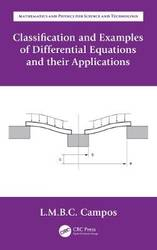Classification and Examples of Differential Equations and their Applications is the sixth book within Ordinary Differential Equations with Applications to Trajectories and Vibrations, Six-volume Set. As a set, they are the fourth volume in the series Mathematics and Physics Applied to Science and Technology. This sixth book consists of one ...
Classification and Examples of Differential Equations and their Applications
Classification and Examples of Differential Equations and their Applications is the sixth book within Ordinary Differential Equations with Applications to Trajectories and Vibrations, Six-volume Set. As a set, they are the fourth volume in the series Mathematics and Physics Applied to Science and Technology. This sixth book consists of one chapter (chapter 10 of the set). It contains 20 examples related to the preceding five books and chapters 1 to 9 of the set. It includes two recollections: the first with a classification of differential equations into 500 standards and the second with a list of 500 applications. The ordinary differential equations are classified in 500 standards concerning methods of solution and related properties, including: (i) linear differential equations with constant or homogeneous coefficients and finite difference equations; (ii) linear and non-linear single differential equations and simultaneous systems; (iii) existence, unicity and other properties; (iv) derivation of general, particular, special, analytic, regular, irregular, and normal integrals; (v) linear differential equations with variable coefficients including known and new special functions. The theory of differential equations is applied to the detailed solution of 500 physical and engineering problems including: (i) one- and multidimensional oscillators, with damping or amplification, with non-resonant or resonant forcing; (ii) single, non-linear, and parametric resonance; (iii) bifurcations and chaotic dynamical systems; (iv) longitudinal and transversal deformations and buckling of bars, beams, and plates; (v) trajectories of particles; (vi) oscillations and waves in non-uniform media, ducts, and wave guides. Provides detailed solution of examples of differential equations of the types covered in tomes l-5 of the set (Ordinary Differential Equations with Applications to Trajectories and Vibrations, Six -volume Set) Includes physical and engineering problems that extend those presented in the tomes 1-6 (Ordinary Differential Equations with Applications to Trajectories and Vibrations, Six-volume Set) Includes a classification of ordinary differential equations and their properties into 500 standards that can serve as a look-up table of methods of solution Covers a recollection of 500 physical and engineering problems and sub-cases that involve the solution of differential equations Presents the problems used as examples including formulation, solution, and interpretation of results
124.58 USD

## Classification and Examples of Differential Equations and their Applications

by Luis Manuel Braga Da Costa Campos
Hardback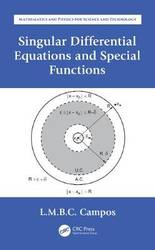Singular Differential Equations and Special Functions is the fifth book within Ordinary Differential Equations with Applications to Trajectories and Vibrations, Six-volume Set. As a set they are the fourth volume in the series Mathematics and Physics Applied to Science and Technology. This fifth book consists of one chapter (chapter 9 ...
Singular Differential Equations and Special Functions
Singular Differential Equations and Special Functions is the fifth book within Ordinary Differential Equations with Applications to Trajectories and Vibrations, Six-volume Set. As a set they are the fourth volume in the series Mathematics and Physics Applied to Science and Technology. This fifth book consists of one chapter (chapter 9 of the set). The chapter starts with general classes of differential equations and simultaneous systems for which the properties of the solutions can be established 'a priori', such as existence and unicity of solution, robustness and uniformity with regard to changes in boundary conditions and parameters, and stability and asymptotic behavior. The book proceeds to consider the most important class of linear differential equations with variable coefficients, that can be analytic functions or have regular or irregular singularities. The solution of singular differential equations by means of (i) power series; (ii) parametric integral transforms; and (iii) continued fractions lead to more than 20 special functions; among these is given greater attention to generalized circular, hyperbolic, Airy, Bessel and hypergeometric differential equations, and the special functions that specify their solutions. Includes existence, unicity, robustness, uniformity, and other theorems for non-linear differential equations Discusses properties of dynamical systems derived from the differential equations describing them, using methods such as Liapunov functions Includes linear differential equations with periodic coefficients, including Floquet theory, Hill infinite determinants and multiple parametric resonance Details theory of the generalized Bessel differential equation, and of the generalized, Gaussian, confluent and extended hypergeometric functions and relations with other 20 special functions Examines Linear Differential Equations with analytic coefficients or regular or irregular singularities, and solutions via power series, parametric integral transforms, and continued fractions
124.58 USD

## Singular Differential Equations and Special Functions

by Luis Manuel Braga Da Costa Campos
Hardback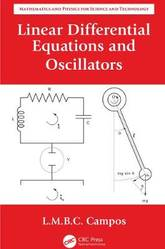Linear Differential Equations and Oscillators is the first book within Ordinary Differential Equations with Applications to Trajectories and Vibrations, Six-volume Set. As a set, they are the fourth volume in the series Mathematics and Physics Applied to Science and Technology. This first book consists of chapters 1 and 2 of ...
Linear Differential Equations and Oscillators
Linear Differential Equations and Oscillators is the first book within Ordinary Differential Equations with Applications to Trajectories and Vibrations, Six-volume Set. As a set, they are the fourth volume in the series Mathematics and Physics Applied to Science and Technology. This first book consists of chapters 1 and 2 of the fourth volume. The first chapter covers linear differential equations of any order whose unforced solution can be obtained from the roots of a characteristic polynomial, namely those: (i) with constant coefficients; (ii) with homogeneous power coefficients with the exponent equal to the order of derivation. The method of characteristic polynomials is also applied to (iii) linear finite difference equations of any order with constant coefficients. The unforced and forced solutions of (i,ii,iii) are examples of some general properties of ordinary differential equations. The second chapter applies the theory of the first chapter to linear second-order oscillators with one degree-of-freedom, such as the mechanical mass-damper-spring-force system and the electrical self-resistor-capacitor-battery circuit. In both cases are treated free undamped, damped, and amplified oscillations; also forced oscillations including beats, resonance, discrete and continuous spectra, and impulsive inputs. Describes general properties of differential and finite difference equations, with focus on linear equations and constant and some power coefficients Presents particular and general solutions for all cases of differential and finite difference equations Provides complete solutions for many cases of forcing including resonant cases Discusses applications to linear second-order mechanical and electrical oscillators with damping Provides solutions with forcing including resonance using the characteristic polynomial, Green' s functions, trigonometrical series, Fourier integrals and Laplace transforms
124.58 USD

## Linear Differential Equations and Oscillators

by Luis Manuel Braga Da Costa Campos
Hardback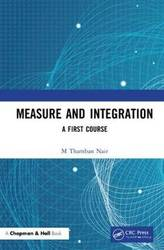This concise text is intended as an introductory course in measure and integration. It covers essentials of the subject, providing ample motivation for new concepts and theorems in the form of discussion and remarks, and with many worked-out examples. The novelty of Measure and Integration: A First Course is in ...
Measure and Integration: A First Course
This concise text is intended as an introductory course in measure and integration. It covers essentials of the subject, providing ample motivation for new concepts and theorems in the form of discussion and remarks, and with many worked-out examples. The novelty of Measure and Integration: A First Course is in its style of exposition of the standard material in a student-friendly manner. New concepts are introduced progressively from less abstract to more abstract so that the subject is felt on solid footing. The book starts with a review of Riemann integration as a motivation for the necessity of introducing the concepts of measure and integration in a general setting. Then the text slowly evolves from the concept of an outer measure of subsets of the set of real line to the concept of Lebesgue measurable sets and Lebesgue measure, and then to the concept of a measure, measurable function, and integration in a more general setting. Again, integration is first introduced with non-negative functions, and then progressively with real and complex-valued functions. A chapter on Fourier transform is introduced only to make the reader realize the importance of the subject to another area of analysis that is essential for the study of advanced courses on partial differential equations. Key Features Numerous examples are worked out in detail. Lebesgue measurability is introduced only after convincing the reader of its necessity. Integrals of a non-negative measurable function is defined after motivating its existence as limits of integrals of simple measurable functions. Several inquisitive questions and important conclusions are displayed prominently. A good number of problems with liberal hints is provided at the end of each chapter. The book is so designed that it can be used as a text for a one-semester course during the first year of a master's program in mathematics or at the senior undergraduate level. About the Author M. Thamban Nair is a professor of mathematics at the Indian Institute of Technology Madras, Chennai, India. He was a post-doctoral fellow at the University of Grenoble, France through a French government scholarship, and also held visiting positions at Australian National University, Canberra, University of Kaiserslautern, Germany, University of St-Etienne, France, and Sun Yat-sen University, Guangzhou, China. The broad area of Prof. Nair's research is in functional analysis and operator equations, more specifically, in the operator theoretic aspects of inverse and ill-posed problems. Prof. Nair has published more than 70 research papers in nationally and internationally reputed journals in the areas of spectral approximations, operator equations, and inverse and ill-posed problems. He is also the author of three books: Functional Analysis: A First Course (PHI-Learning, New Delhi), Linear Operator Equations: Approximation and Regularization (World Scientific, Singapore), and Calculus of One Variable (Ane Books Pvt. Ltd, New Delhi), and he is also co-author of Linear Algebra (Springer, New York).
139.46 USD

## Measure and Integration: A First Course

by M Thamban Nair
Hardback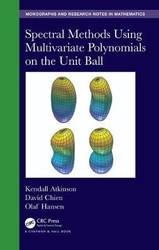Spectral Methods Using Multivariate Polynomials on the Unit Ball is a research level text on a numerical method for the solution of partial differential equations. The authors introduce, illustrate with examples, and analyze 'spectral methods' that are based on multivariate polynomial approximations. The method presented is an alternative to finite ...
Spectral Methods Using Multivariate Polynomials On The Unit Ball
Spectral Methods Using Multivariate Polynomials on the Unit Ball is a research level text on a numerical method for the solution of partial differential equations. The authors introduce, illustrate with examples, and analyze 'spectral methods' that are based on multivariate polynomial approximations. The method presented is an alternative to finite element and difference methods for regions that are diffeomorphic to the unit disk, in two dimensions, and the unit ball, in three dimensions. The speed of convergence of spectral methods is usually much higher than that of finite element or finite difference methods. Features Introduces the use of multivariate polynomials for the construction and analysis of spectral methods for linear and nonlinear boundary value problems Suitable for researchers and students in numerical analysis of PDEs, along with anyone interested in applying this method to a particular physical problem One of the few texts to address this area using multivariate orthogonal polynomials, rather than tensor products of univariate polynomials.
251.06 USD

## Spectral Methods Using Multivariate Polynomials On The Unit Ball

by Olaf Hansen, David Chien, Kendall Atkinson
Hardback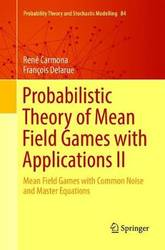This two-volume book offers a comprehensive treatment of the probabilistic approach to mean field game models and their applications. The book is self-contained in nature and includes original material and applications with explicit examples throughout, including numerical solutions. Volume II tackles the analysis of mean field games in which the ...
Probabilistic Theory of Mean Field Games with Applications II: Mean Field Games with Common Noise and Master Equations
This two-volume book offers a comprehensive treatment of the probabilistic approach to mean field game models and their applications. The book is self-contained in nature and includes original material and applications with explicit examples throughout, including numerical solutions. Volume II tackles the analysis of mean field games in which the players are affected by a common source of noise. The first part of the volume introduces and studies the concepts of weak and strong equilibria, and establishes general solvability results. The second part is devoted to the study of the master equation, a partial differential equation satisfied by the value function of the game over the space of probability measures. Existence of viscosity and classical solutions are proven and used to study asymptotics of games with finitely many players. Together, both Volume I and Volume II will greatly benefit mathematical graduate students and researchers interested in mean field games. The authors provide a detailed road map through the book allowing different access points for different readers and building up the level of technical detail. The accessible approach and overview will allow interested researchers in the applied sciences to obtain a clear overview of the state of the art in mean field games.
156.450000 USD

## Probabilistic Theory of Mean Field Games with Applications II: Mean Field Games with Common Noise and Master Equations

by Francois Delarue, Rene Carmona
Paperback / softback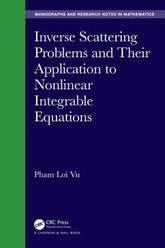Inverse Scattering Problems and Their Application to Nonlinear Integrable Equations is devoted to inverse scattering problems (ISPs) for differential equations and their application to nonlinear evolution equations (NLEEs). The book is suitable for anyone who has a mathematical background and interest in functional analysis, partial differential equations, equations of mathematical ...
Inverse Scattering Problems and Their Application to Nonlinear Integrable Equations
Inverse Scattering Problems and Their Application to Nonlinear Integrable Equations is devoted to inverse scattering problems (ISPs) for differential equations and their application to nonlinear evolution equations (NLEEs). The book is suitable for anyone who has a mathematical background and interest in functional analysis, partial differential equations, equations of mathematical physics, and functions of a complex variable. This book is intended for a wide community working with inverse scattering problems and their applications; in particular, there is a traditional community in mathematical physics. In this monograph, the problems are solved step-by-step, and detailed proofs are given for the problems to make the topics more accessible for students who are approaching them for the first time. Features * The unique solvability of ISPs are proved. The scattering data of the considered inverse scattering problems (ISPs) are described completely. * Solving the associated initial value problem or initial-boundary value problem for the nonlinear evolution equations (NLEEs) is carried out step-by-step. Namely, the NLEE can be written as the compatibility condition of two linear equations. The unknown boundary values are calculated with the help of the Lax (generalized) equation, and then the time-dependent scattering data (SD) are constructed from the initial and boundary conditions. * The potentials are recovered uniquely in terms of time-dependent SD, and the solution of the NLEEs is expressed uniquely in terms of the found solutions of the ISP. * Since the considered ISPs are solved well, then the SPs generated by two linear equations constitute the inverse scattering method (ISM). The application of the ISM to solving the NLEEs is consistent and is effectively embedded in the schema of the ISM.
189.000000 USD

## Inverse Scattering Problems and Their Application to Nonlinear Integrable Equations

by Pham Loi Vu
Hardback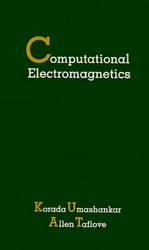A self-study tutorial which presents the fundamental principles and rigorous numerical validations of a major contemporary branch in frequency-domain computational electromagnetics.
Computational Electromagnetics
A self-study tutorial which presents the fundamental principles and rigorous numerical validations of a major contemporary branch in frequency-domain computational electromagnetics.
225.750000 USD

## Computational Electromagnetics

Hardback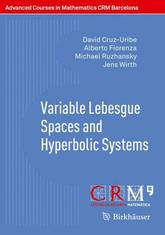This book targets graduate students and researchers who want to learn about Lebesgue spaces and solutions to hyperbolic equations. It is divided into two parts. Part 1 provides an introduction to the theory of variable Lebesgue spaces: Banach function spaces like the classical Lebesgue spaces but with the constant exponent ...
Variable Lebesgue Spaces and Hyperbolic Systems
This book targets graduate students and researchers who want to learn about Lebesgue spaces and solutions to hyperbolic equations. It is divided into two parts. Part 1 provides an introduction to the theory of variable Lebesgue spaces: Banach function spaces like the classical Lebesgue spaces but with the constant exponent replaced by an exponent function. These spaces arise naturally from the study of partial differential equations and variational integrals with non-standard growth conditions. They have applications to electrorheological fluids in physics and to image reconstruction. After an introduction that sketches history and motivation, the authors develop the function space properties of variable Lebesgue spaces; proofs are modeled on the classical theory. Subsequently, the Hardy-Littlewood maximal operator is discussed. In the last chapter, other operators from harmonic analysis are considered, such as convolution operators and singular integrals. The text is mostly self-contained, with only some more technical proofs and background material omitted. Part 2 gives an overview of the asymptotic properties of solutions to hyperbolic equations and systems with time-dependent coefficients. First, an overview of known results is given for general scalar hyperbolic equations of higher order with constant coefficients. Then strongly hyperbolic systems with time-dependent coefficients are considered. A feature of the described approach is that oscillations in coefficients are allowed. Propagators for the Cauchy problems are constructed as oscillatory integrals by working in appropriate time-frequency symbol classes. A number of examples is considered and the sharpness of results is discussed. An exemplary treatment of dissipative terms shows how effective lower order terms can change asymptotic properties and thus complements the exposition.
36.740000 USD

## Variable Lebesgue Spaces and Hyperbolic Systems

by Jens Wirth, Michael Ruzhansky, Alberto Fiorenza, David V. Cruz-Uribe
Paperback / softback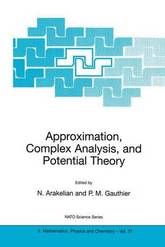Hermann Weyl considered value distribution theory to be the greatest mathematical achievement of the first half of the 20th century. The present lectures show that this beautiful theory is still growing. An important tool is complex approximation and some of the lectures are devoted to this topic. Harmonic approximation started ...
Approximation, Complex Analysis, and Potential Theory
Hermann Weyl considered value distribution theory to be the greatest mathematical achievement of the first half of the 20th century. The present lectures show that this beautiful theory is still growing. An important tool is complex approximation and some of the lectures are devoted to this topic. Harmonic approximation started to flourish astonishingly rapidly towards the end of the 20th century, and the latest development, including approximation manifolds, are presented here. Since de Branges confirmed the Bieberbach conjecture, the primary problem in geometric function theory is to find the precise value of the Bloch constant. After more than half a century without progress, a breakthrough was recently achieved and is presented. Other topics are also presented, including Jensen measures. A valuable introduction to currently active areas of complex analysis and potential theory. Can be read with profit by both students of analysis and research mathematicians.